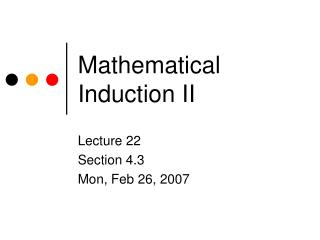DownloadDownload PresentationMathematical Induction II

# Mathematical Induction II

Télécharger la présentation## Mathematical Induction II

- - - - - - - - - - - - - - - - - - - - - - - - - - - E N D - - - - - - - - - - - - - - - - - - - - - - - - - - -
##### Presentation Transcript

1. Mathematical Induction II Lecture 22 Section 4.3 Mon, Feb 26, 2007

2. Example • Find a formula for 1 + 3 + 5 + … + (2n – 1). and use mathematical induction to prove that it is correct.

3. Exercise • Find a formula for 12 + 32 + 52 + … + (2n – 1)2. and use mathematical induction to prove that it is correct.

4. Example: Binary Search Trees • In a complete binary search tree of depth n - 1, (n rows) what is the average number of comparisons required to locate an element? (Assuming all positions are equally likely.) • Analysis • Elements in row 1 require 1 comparison. • Elements in row 2 require 2 comparisons. • Elements in row 3 require 3 comparisons. • In general, elements in row k require k comparisons.

5. Example: Binary Search Trees • Further analysis • Row 1 contains 1 element. • Row 2 contains 2 elements. • Row 3 contains 4 elements. • In general, row k contains 2k - 1 elements. • Fact • The average number of comparisons is the total number of comparisons required for all elements divided by the number of elements.

6. Example: Binary Search Trees • The total number of elements is 1 + 2 + 4 + 8 + … + 2n – 1. • The total number of comparisons is 11 + 22 + 34 + 48 + … + n2n – 1. • Therefore, the average is

7. Example: Binary Search Trees • Prove by mathematical induction that 1 + 2 + 4 + 8 + … + 2n – 1 = 2n – 1. • Prove by mathematical induction that 11 + 22 + 34 + 48 + … + n2n – 1 = (n – 1)2n + 1. • Then, the average is • This is approximately equal to n – 1 (for large n).

8. Example: Recursive Sequences • Define a sequence a1 = 2, an = 2an – 1 – 1 for n  2. • Find a non-recursive formula for an and prove that it is correct. • Analysis • {an} = {2, 3, 5, 9, 17, 33, …}. • {an – 1} = {1, 2, 4, 8, 16, 32, …}. • Conjecture that an = 2n – 1 + 1.

9. Example: Recursive Sequences • Proof: • Basic Step • P(1) is true since a1 = 2 = 20 + 1. • Inductive Step • Suppose that ak = 2k – 1 + 1 for some k 1.

10. Example: Recursive Sequences • Then ak + 1 = 2ak – 1 (by def.) = 2(2k – 1 + 1) – 1 (by ind. hyp.) = 2k + 2 – 1 = 2k + 1. • Therefore, P(k + 1) is true. • Therefore, an = 2n + 1 for all n 1.

11. Example • Define {an} as • a1 = 2, • an = 3an – 1 + 1, for all n 2. • Find a non-recursive formula for an and prove that it is correct using mathematical induction.